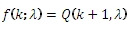# SQL Server POISSON.INV function

POISSONINV

Updated: 31 July 2010

Use POISSONINV to calculate the inverse of the cumulative distribution function of the Poisson distribution.

The formula for the cumulative distribution function is:Syntax
SELECT [wctStatistics].[wct].[POISSONINV] (
<@P, float,>
,<@Mean, float,>)
Arguments
@P
the cumulative distribution function. @P is an expression of type float or of a type that implicitly converts to float
@Mean
is the mean of the distribution. @Mean is an expression of type float or of a type that implicitly converts to float
Return Types
float
Remarks
·         @P must be greater than zero and less than one (0 < @P < 1).
·         @Mean must be greater than or equal to zero (0 ≤ @Mean).
Examples
Calculate the cumulative distribution function:
SELECT wct.POISSON(2,5,'True')
This produces the following result
----------------------
0.124652019483081

(1 row(s) affected)

Calculate the inverse:
SELECT wct.POISSONINV(0.124652019483081, 5)
This produces the following result
----------------------
2

(1 row(s) affected)

### SupportCopyright 2008-2021 Westclintech LLC         Privacy Policy        Terms of Service